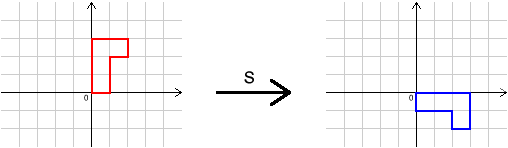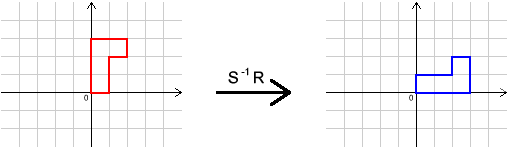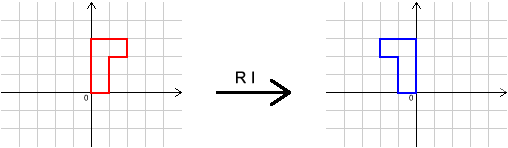#### You may also like### Chess

What would be the smallest number of moves needed to move a Knight from a chess set from one corner to the opposite corner of a 99 by 99 square board?### 2001 Spatial Oddity

With one cut a piece of card 16 cm by 9 cm can be made into two pieces which can be rearranged to form a square 12 cm by 12 cm. Explain how this can be done.### Screwed-up

A cylindrical helix is just a spiral on a cylinder, like an ordinary spring or the thread on a bolt. If I turn a left-handed helix over (top to bottom) does it become a right handed helix?

# Decoding Transformations

##### Age 11 to 14 Challenge Level:

In this question, each of the letters $I$, $R$, $S$ and $T$ represents a different transformation.

We can do one transformation followed by another. For example, $R S$ means do $R$, then $S$''.

We can also undo transformations. For example, $R^{-1}$ means do the inverse (opposite) of $R$''.

Here are the effects of some transformations on a shape. Can you describe the transformations $I$, $R$, $S$ and $T$?What single transformation has the same effect as $R S T I R^{-1}S^{-1}T^{-1}I^{-1}$?

This problem is the first of three related problems.
The follow-up problems are Combining Transformations and Simplifying Transformations .